# Bigger cycles in cubic graphs (Solved)

 Importance: Medium ✭✭
 Author(s):
 Subject: Graph Theory » Basic Graph Theory » » Cycles
 Keywords: cubic cycle
 Recomm. for undergrads: no
 Posted by: mdevos on: August 31st, 2007
 Solved by: See Stable dominating circuits in snarks" (Discrete Math 233 (247-256) 2001) by Martin Kochhol
Problem   Letbe a cyclically 4-edge-connected cubic graph and letbe a cycle of. Must there exist a cycleso that?

The main interest in this problem is that an affirmative solution would imply the Cycle double cover conjecture. The idea behind this is an old one - probably due to Seymour - which shall be described below. I (M. DeVos) regard this problem as folklore, which is why I haven't listed an author.

One sensible approach to the cycle double cover conjecture is that described in Faithful cycle covers. Quickly, the idea here is to consider a more general problem.. given a graphand a map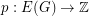, say that a list of cycles ofis a-cover if every edgeappears in exactly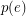cycles from the list. Now, we are interested in what properties guarantee the existence of a-cover. The Cycle double cover conjecture asserts that a-cover exists wheneveris bridgeless andis constantly 2. Another interesting special case is whenis a bridgeless cubic graph, the range ofis a subset of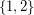, and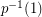is a union of (edge sets of) cycles. It might be tempting to hope that under these assumptions a-cover would always exist, but this is false.. ifis the Petersen graph andhas weight 2 on a perfect matching and 1 elsewhere, then there is no-cover. In this weighting, the edges of weight 1 form two cycles. The following conjecture asserts that no such example exists if the edges of weight 1 form a single cycle.

Conjecture   Letbe a bridgeless cubic graph, let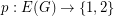and assume thatis a cycle. Then there exists a-cover of.

This conjecture is equivalent to the assertion that for every bridgeless cubic graphand every cycleof, there exists a cycle double cover ofusing. So, in particular, it implies the cycle double cover conjecture. An affirmative solution to the main problem on this page would imply this conjecture (and thus cycle double cover). Let us ignore the details about edge connectivity (the ideas here are standard - and easy to find) and state the main idea behind this implication. The trick is to proceed by induction (say on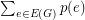), then choose a cycleso that, and consider(symmetric difference). Note thatis a union of cycles (which we will eventually use in our cover). Next, modifyandto form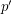andby decreasing the weight of every edge inand then deleting edges of weight 0. An easy check shows thatis a subdivision of a bridgeless cubic graph, andis a weight function onwhich has weight 1 on. So, by induction, we find a-cover of. Now adding back the cycles formed bygives us a-cover of.

Although the problem looks a bit greedy, it is true in a number of special cases. First off, ifis a Hamiltonian cycle of, then Smith's theorem shows thathas another Hamiltonian cycle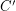(which obviously satisfies the condition). A somewhat more subtle application of the same theorem shows that the problem also has an affirmative answer when. If we drop the assumption of cyclic 4-edge-connectivity, there is a counterexample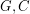where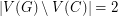which is based on gluing two Petersen graphs together at a vertex. However, as far as we know, the only such examples have nontrivial 3-edge-cuts.

### This conjecture is false

In the paper "Stable dominating circuits in snarks" (Discrete Math 233 (247-256) 2001) Martin Kochhol gives a counter example to this conjecture (in fact he gives an infinite family of them).

Using computer search I have found even smaller counter examples (the smallest has just 20 vertices).

Best regards,

Jonas Hägglund

## Comment viewing options

Select your preferred way to display the comments and click "Save settings" to activate your changes.